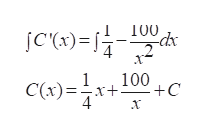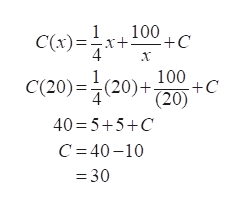The DeWitt Company has found that the rate of change of its average cost for product is C'(x)=(1/4)-(100/x^2)where x is the number of units and cost is in dollars. The average cost of producing 20 units is \$40.00.(a) Find the average cost function for the product.(b) Find the average cost of 50 units of the product.

Question

The DeWitt Company has found that the rate of change of its average cost for product is C'(x)=(1/4)-(100/x^2)

where x is the number of units and cost is in dollars. The average cost of producing 20 units is \$40.00.

(a) Find the average cost function for the product.

(b) Find the average cost of 50 units of the product.

Step 1

Given information:

The DeWitt Company has found that the rate of change of its average cost for product is

Step 2

Calculation:

Integrate the function C\\\'(x) with respect to x, we gethelp_outlineImage TranscriptioncloseT00 jC'(x) 4 1 C(x)x 100 +C 4 fullscreen
Step 3

It is given that the average cost of producing 20 units ...help_outlineImage Transcriptionclose100 +C C(x) 4 100 +C (20) C(20) (20)+ 40 5+5+C C 40-10 =30 fullscreen

Want to see the full answer?

See Solution

Want to see this answer and more?

Our solutions are written by experts, many with advanced degrees, and available 24/7

See Solution
Tagged in

Integration